4WX Internet Search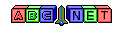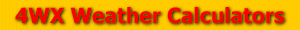# Station Pressure

From the user, a station elevation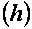and an altimeter setting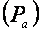are given. Before calculating the station pressure, the station elevation must be converted to meters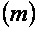using the formula below: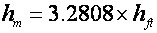Also, the altimeter setting must be converted to inches of mercury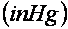For information on how to convert pressure, use the link below:

Then, the station pressure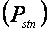can be calculated using the formula below:Then, the station pressure can be converted to other pressure units, using the link above.

Back to 4WX Weather Calculators index page

4WX Calculators Credit and disclaimer